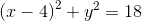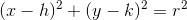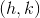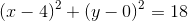# SAT II Math II : Symmetry

## Example Questions

### Example Question #1 : Symmetry

Which of the following symmetries applies to the graph of the relation?

I) Symmetry with respect to the origin

II) Symmetry with respect to the-axis

III) Symmetry with respect to the-axis

III only

I only

None of these

II only

I, II, and III

II only

Explanation:

The relationis a circle with centerand radius.

In other words, it is a circle with center at the origin, translated rightunits and upunits.oris a circle translated right 4 units and up zero units. The upshot is that the circle moves along the-axis only, and therefore is symmetric with respect to the-axis, but not the-axis. Also, as a consequence, it is not symmetric with respect to the origin.

### All SAT II Math II Resources